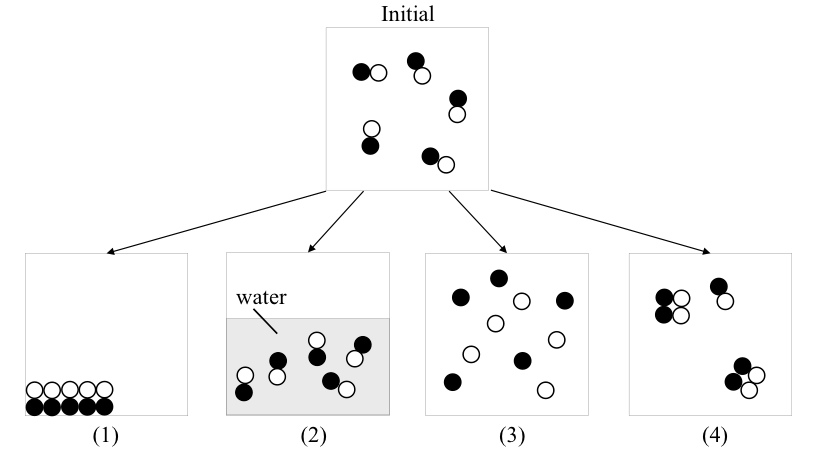# Problem: Consider the following figure of four processes from the initial state. The substances are in gas phase initially. Which process(es) would result in an entropy increase?   A. (1) and (2)  B. (1) and (3) C. only (3) D. (1), (2), and (4) E. only (4)

###### FREE Expert Solution
100% (282 ratings)
###### Problem Details

Consider the following figure of four processes from the initial state. The substances are in gas phase initially. Which process(es) would result in an entropy increase?

A. (1) and (2)

B. (1) and (3)

C. only (3)

D. (1), (2), and (4)

E. only (4)Frequently Asked Questions

What scientific concept do you need to know in order to solve this problem?

Our tutors have indicated that to solve this problem you will need to apply the Entropy concept. You can view video lessons to learn Entropy. Or if you need more Entropy practice, you can also practice Entropy practice problems.

What is the difficulty of this problem?

Our tutors rated the difficulty ofConsider the following figure of four processes from the ini...as medium difficulty.

How long does this problem take to solve?

Our expert Chemistry tutor, Jules took 2 minutes and 32 seconds to solve this problem. You can follow their steps in the video explanation above.